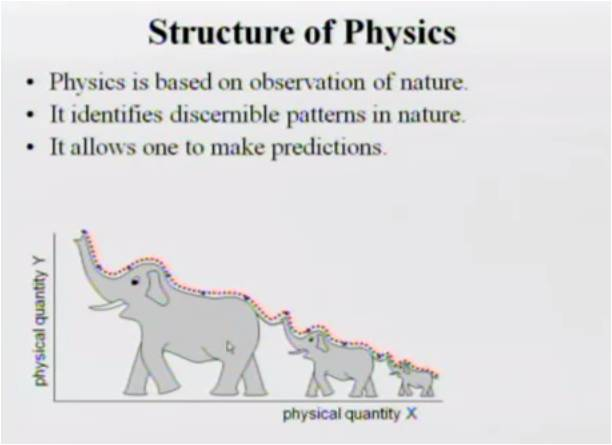# InfoCoBuild

## Physics 240 - Physics for Science and Engineering I

Physics 240: Physics for Science and Engineering I (Calculus-based Mechanics) (UMKC). Taught by Professor Jerzy Wrobel, this course provides an introduction to mechanics, wave motion and sound and heat and thermodynamics. And the course is based on the 8th edition of a textbook Physics for Scientists and Engineers authored R.A. Serway & J.W. Jewett, Jr.Lecture 01 - Review of the course syllabus Lecture 02 - Introduction to Physics Lecture 03 - Scalar Quantities Lecture 04 - Area, Volume and Density Lecture 05 - Vectors Lecture 06 - Scalar Product and Cartesian Coordinate System Lecture 07 - Vector Quantities Lecture 08 - Velocity and Acceleration Lecture 09 - Motion with Constant Velocity Lecture 10 - Motion with Constant Acceleration Lecture 11 - Inverse Relations Lecture 12 - Galilean Transformation Lecture 13 - Laws of Motion; the concept of force Lecture 14 - Laws of Motion; four fundamental interactions Lecture 15 - Laws of Motion; weight, normal force, static friction Lecture 16 - Laws of Motion; kinetic friction, tension, drag Lecture 17 - Uniform Circular Motion Lecture 18 - Inertial Forces Lecture 19 - Inertial Forces (cont.) Lecture 20 - Newton's Second Law in a non-Inertial Reference Frame Lecture 21 - The Work-Energy Theorem (v.1) Lecture 22 - Power, Conservative Interaction, Potential Energy Lecture 23 - Mechanical Energy Lecture 24 - Applications of the Work-Energy Theorem Lecture 25 - Relation between Descriptions of Interaction and Descriptions of Motion Lecture 26 - Impulse-Momentum Theorem Lecture 27 - Conservation of Total Momentum Lecture 28 - Center of Mass Lecture 29 - Newton's Second Law, Center of Mass, Kinetic Energy Lecture 30 - Work-Energy Theorem IV; Total Kinetic Energy Lecture 31 - Work-Energy Theorem; Gravitational Interaction Lecture 32 - Angular Momentum and Torque Lecture 33 - Center of Force and Rigid Body Lecture 34 - Total Angular Momentum of a Rigid Body Lecture 35 - Motion of Center of Mass, Axis of Rotation, Moment of Inertia Lecture 36 - Kinetic Energy of a Rigid Body Lecture 37 - Application of Newton's Second Law Lecture 38 - Application of Newton's Second Law for Analysis Lecture 39 - Internal Interactions Lecture 40 - Hooke's Law Lecture 41 - Liquid in Equilibrium Lecture 42 - Archimedes Principle and Bernoulli's Equation Lecture 43 - Motion of Particles; Sinusoidal Waves Lecture 43 - Concept of Heat Transfer Lecture 44 - Heat Capacity Lecture 45 - Thermodynamic Processes Lecture 46 - The Firs Law of Thermodynamics Lecture 47 - Microscopic Model of an Ideal Gas Lecture 48 - Entropy Lecture 49 - Second Law of Thermodynamics Lecture 50 - Consequences of the Second Law of Thermodynamics Lecture 51 - Review of Concepts